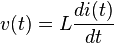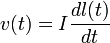The paraformer is a particular type of transformer. It transfers the power from primary to secondary windings not by mutual inductance coupling but by a variation of a parameter in its magnetic circuit. First described by Wanlass, et al., 1968.

Assuming Faraday's law of induction,$v(t) = L \frac{di(t)}{dt}$

it is possible to obtain a voltage at the secondary winding terminals also thanks to a variation of the inductance, so that$v(t) = I \frac{dl(t)}{dt}$

This can be accomplished by for example modulating the saturation of the core by means of an applied variable magnetic field. It works even if primary and secondary windings magnetic coupling is zero (when the fluxes are mutually orthogonal).